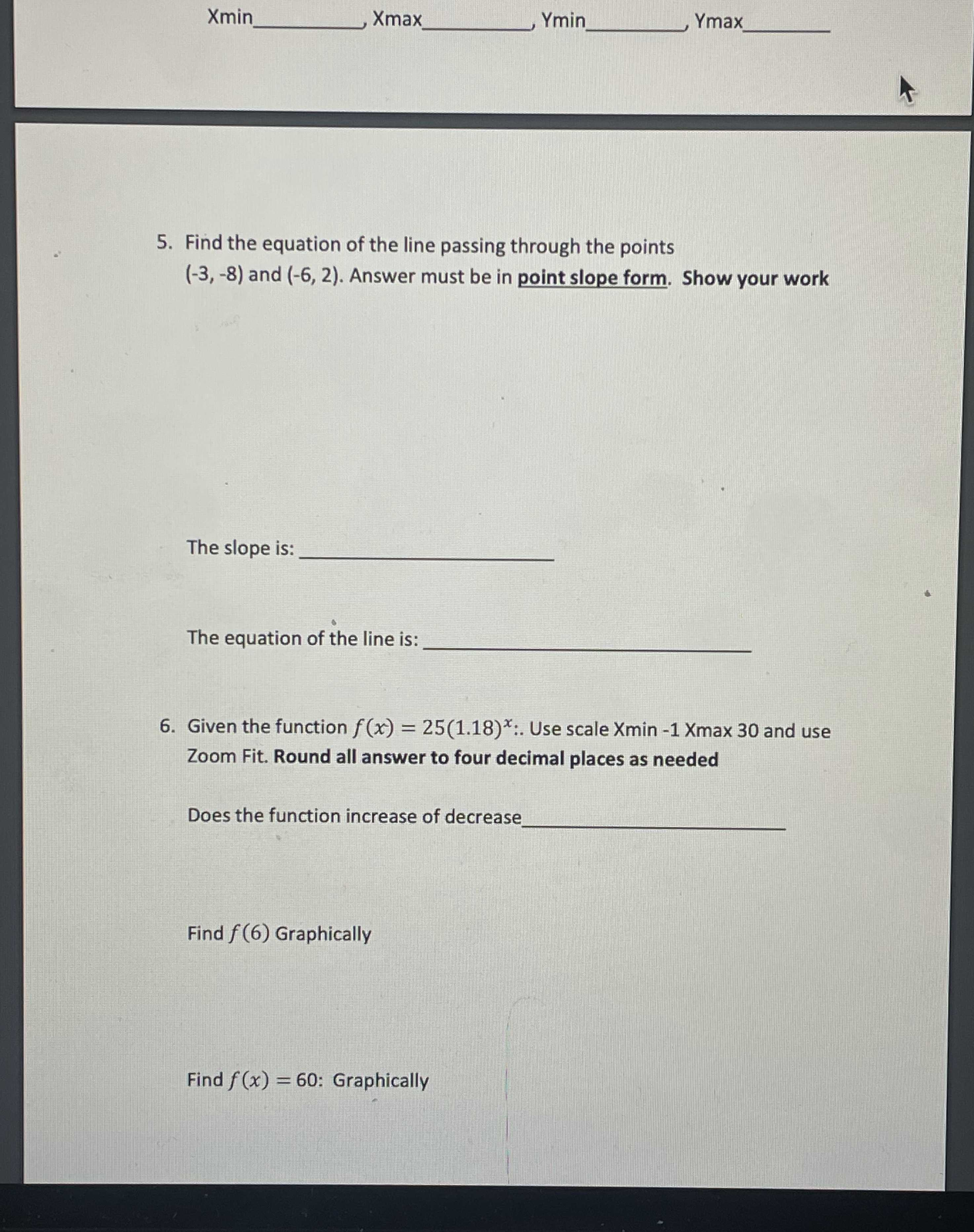### ¿Todavía tienes preguntas de matemáticas?

Pregunte a nuestros tutores expertos
Algebra
Pregunta5. Find the equation of the line passing through the points $$( - 3 , - 8 )$$ and $$( - 6,2 )$$ . Answer must be in point slope form. Show your work.The slope is: The equation of the line is:

6. Given the function $$f ( x ) = 25 ( 1.18 ) ^ { x } :$$ Use scale Xmin $$- 1$$ Xmax $$30$$ and use Zoom Fit. Round all answer to four decimal places as needed Does the function increase of decrease.

Find $$f ( 6 )$$ Graphically Find $$f ( x ) = 60 :$$ Graphically

5.

Fraction Form

$$y = - \frac { 10 } { 3 } x - 18$$

6.

increasing function

850.3

1.5

Solución
View full explanation on CameraMath App.